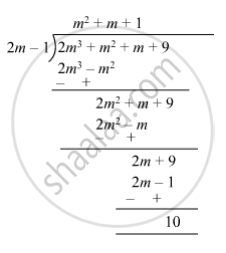SSC (English Medium) Class 8Maharashtra State Board
Share

# Divide and Write the Quotient and the Remainder. (2m3 + M2 + M + 9) ÷ (2m − 1) - SSC (English Medium) Class 8 - Mathematics

ConceptTo Divide a Polynomial by a Binomial

#### Question

Divide and write the quotient and the remainder.

(2m3 + m2 + m + 9) ÷ (2m − 1)

#### Solution

(2m3 + m2 + m + 9) ÷ (2m − 1)So, quotient = $m^2 + m + 1$ and remainder = 10.

Is there an error in this question or solution?

#### APPEARS IN

Balbharati Solution for Balbharati Class 8 Mathematics (2019 to Current)
Chapter 10: Division of Polynomials
Practice Set 10.2 | Q: 4 | Page no. 66
Solution Divide and Write the Quotient and the Remainder. (2m3 + M2 + M + 9) ÷ (2m − 1) Concept: To Divide a Polynomial by a Binomial.
S